Home > CC2MN > Chapter cc27 > Lesson cc27.1.3 > Problem7-34

7-34.
1. Copy and simplify the following expressions by combining like terms, making zeros, and using the Distributive Property. Using algebra tiles may be helpful. 7-34 HW eTool (CPM). Homework Help ✎

1. (–1) + 4x + 2 + 2x + x

2. –8x + 4 + (–3) + 10x

3. (–4) + 1x2 + 3x + 4

4. 2(3x – 2)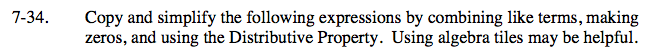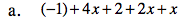Group similar terms together in order to combine like terms.
(4x + 2x + x) + (−1 + 2)

7x + 1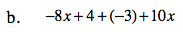Visualize 8 negative x tiles, 4 positive unit tiles, 3 negative unit tiles, and 10 positive x tiles. If a negative and a positive tile of the same value make a zero, how many tiles are remaining?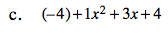Group similar terms together and make zeros.
(−4 + 4) + 1x2 + 3x

Now the fours make a zero.
0 + 1x² + 3x

x2 + 3x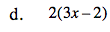Distribute the 2 on the outside to each of the terms inside the parentheses.
2(3x) − 2(2)

Simplify.

Use the eTool below for help with this problem.
Click the link at right for the full version of the eTool: CC2 7-34 HW eTool